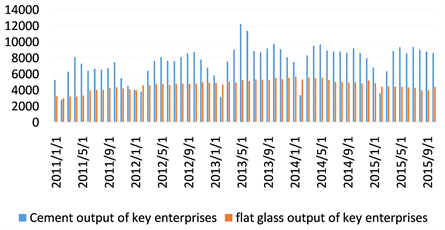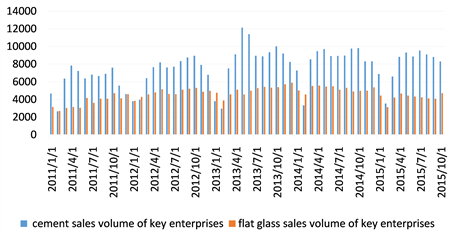﻿ 我国建材行业经济增长和主要产品产销量关系的实证分析

# 我国建材行业经济增长和主要产品产销量关系的实证分析Empirical Analysis on the Relationship between Economic Growth and Production and Sales of Main Products in China’s Building Materials Industry

Abstract: Using the statistical software SPSS, the Cobb-Douglas production function is simulated based on the annual original data of the national building materials industry from 05 to 17 years and the annual data of the non-metallic manufacturing industry from 10 to 17 years, and the growth factors are empirically analyzed. And the building materials’ owners want products (cement, glass) to analyze the relationship between production and sales.

1. 引言

2. 增长模型假设及计算过程

Cobb-Douglas生产函数 $Y=A{K}^{\alpha }{L}^{\beta }$ 经微分、差分替代后得索洛增长速度方程： $y=a+\alpha k+\beta l$   ，其中：y：产出增长速度(水平法计算年平均增长速度，下同)；k：资本增长速度；l：劳动增长速度；a：技术进步增长速度。由上式易得： $1=\frac{a}{y}+\alpha \frac{k}{y}+\beta \frac{l}{y}$ 由此得全要素生产率 $\frac{a}{y}$ ，资本和劳动增长的贡献率分别为 

$Y=A{K}^{\alpha }{L}^{\beta }$ 易得： $\mathrm{ln}Y=\mathrm{ln}A+\mathrm{ln}K+\mathrm{ln}L$ ，便于进行回归分析。产出值一般采用总产值；劳动投入量一般用劳动者人数等指标确定；资金投入量一般用当年固定资产原值与定额流动资金年平均余额之和来确定。

3. 实证分析

3.1. 2005~2017年全国建材行业经济增长分析

$\text{Ln}\left(Y\right)=-6.407+0.013\text{Ln}X1+1.877\text{Ln}X2$Table 1. Regression equation variance analysis tableTable 2. Regression equation coefficient analysis tableTable 3. Analysis of economic growth in China's building materials industry

3.2. 2010~2017年全国建材及非金属矿业选业增长分析

10~17年我国非金属矿业选业的原始数据，对数化，用统计软件SPSS处理后采用强度OLS估计，得到表4表5

$\text{Ln}\left(Y\right)=3.889+0.698\text{Ln}X1-0.26\text{Ln}X2$

α = 0.698、β = −0.26 (正规化后：α = 1.5936，β = −0.5936)。Table 5. Regression equation coefficient table

α + β = 0.438，表明05~17年间，我国非金属矿业选业正处于规模收益递减阶段，即劳动投入量每增加一个单位，资本边际产出量的增加小于一个单位。从目前我国非金属矿业选业的总体状态也能看出，这个行业中，起重大作用的还是靠大规模的机器生产。上述模型分析得出的结论也显得有一定实际意义。Table 6. Analysis of economic growth in national building materials and non-metallic mining industries

3.3. 建材工业重点行业水泥，平板玻璃产销量分析Figure 1. Monthly chart of cement and flat glass productionFigure 2. Cement flat glass monthly market sales table

4. 结论

NOTES

*通讯作者。

 罗伯特∙索罗. 经济增长因素分析[M]. 北京: 商务印书馆, 1999.

 国家建筑材料工业局. 建筑材料工业统计资料汇编[M]. 北京: 中国工业出版社, 1991-1998.

Top